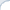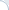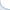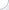There is an opinion that a statistical test is merely a heuristic with good theoretical guarantees. In particular, because, if you take a large enough sample, you are likely to get a statistically significant result. Why? For instance, in the context of information retrieval systems, no two different systems have absolutely identical values of the mean average precision or ERR. A large enough sample would allow us to detect this situation. If a large sample can get us a statistically significant result, is statistical testing useful?

First of all, in the case of one sided tests, adding more data may not lead to statistically significant results. Imagine, that a retrieval system A is better than a retrieval system B. We may have some prior beliefs that B is better than A and, therefore, we try to reject the hypothesis that B is worse than A. Due to high variance in query-specific performance scores, it may be possible to reject this hypothesis for a small set of queries. However, if we take a large enough sample, such rejection would be unlikely.

Let us now consider two-sided tests. In this case, you are likely to "enforce" statistical significance by adding more data. In other words, if systems A and B have slightly different average performance scores, we will able to select a large enough sample of queries to reject the hypothesis that A is the same as B. However, because the sample is large, the difference in average performance scores will be measured very reliably (most of the time). Thus, we will see that the difference between A and B is not substantial. In contrast, if we select a small sample, we may accidentally see a large difference between A and B, but this difference will not be statistically significant.

So, what is the bottom line? Statistical significance may be a heuristic, but, nevertheless, a very important one. If we see a large difference between A and B that is not statistically significant, then the true difference between in average performance between A and B may not be substantial. The large difference observed for a small sample of queries can be due to a high variance in query-specific performance scores. And, if we measure average performance between A and B using a large sample of queries, we may be able to detect a statistically significant difference, but the difference in performance will not be substantial. Or, alternatively, we can save the effort (evaluation can be very costly!) and do something more useful. This would be a benefit of carrying out a statistical test (using a smaller sample).

PS: Another concern related to statistical significance testing is "fishing" for p-values. If you do multiple experiments, you can get a statistically significant result by chance. Sometimes, people just discard all failed experiments and stick with a few tests where, e.g., p-values < 0.05. Ideally, this should not happen: One needs to adjust p-values so that all experiments (in a series of other relevant tests) are taken into account. Some of the adjustments methods are discussed in the previous blog post.